# Built-in plotting methods for Raw objects#

This tutorial shows how to plot continuous data as a time series, how to plot the spectral density of continuous data, and how to plot the sensor locations and projectors stored in `Raw` objects.

As usual we’ll start by importing the modules we need, loading some example data, and cropping the `Raw` object to just 60 seconds before loading it into RAM to save memory:

```import os

import mne

sample_data_folder = mne.datasets.sample.data_path()
sample_data_raw_file = os.path.join(sample_data_folder, 'MEG', 'sample',
'sample_audvis_raw.fif')
```
```Opening raw data file /home/circleci/mne_data/MNE-sample-data/MEG/sample/sample_audvis_raw.fif...
Read a total of 3 projection items:
PCA-v1 (1 x 102)  idle
PCA-v2 (1 x 102)  idle
PCA-v3 (1 x 102)  idle
Range : 25800 ... 192599 =     42.956 ...   320.670 secs
Reading 0 ... 36037  =      0.000 ...    60.000 secs...
```
Measurement date December 03, 2002 19:01:10 GMT MEG Unknown 146 points 204 Gradiometers, 102 Magnetometers, 9 Stimulus, 60 EEG, 1 EOG MEG 2443, EEG 053 EOG 061 Not available 600.61 Hz 0.10 Hz 172.18 Hz PCA-v1 : offPCA-v2 : offPCA-v3 : off sample_audvis_raw.fif 00:01:01 (HH:MM:SS)

We’ve seen in a previous tutorial how to plot data from a `Raw` object using matplotlib, but `Raw` objects also have several built-in plotting methods:

The first one is discussed here in detail; the last two are shown briefly and covered in-depth in other tutorials. This tutorial also covers a few ways of plotting the spectral content of `Raw` data.

## Interactive data browsing with `Raw.plot()`#

The `plot` method of `Raw` objects provides a versatile interface for exploring continuous data. For interactive viewing and data quality checking, it can be called with no additional parameters:

```raw.plot()
```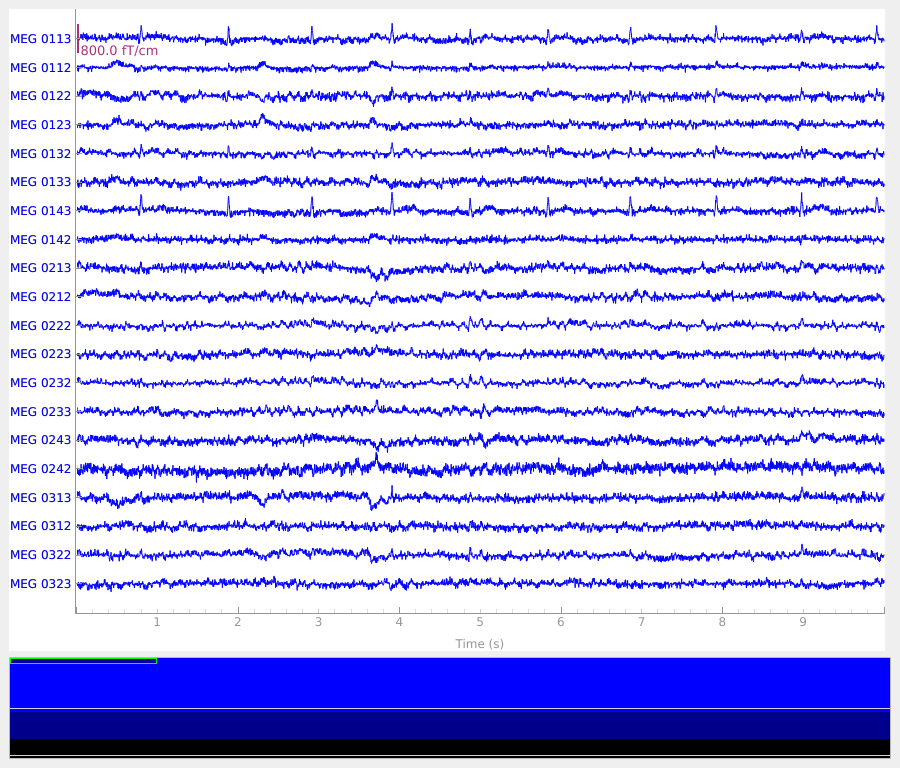It may not be obvious when viewing this tutorial online, but by default, the `plot` method generates an interactive plot window with several useful features:

• It spaces the channels equally along the y-axis.

• 20 channels are shown by default; you can scroll through the channels using the and arrow keys, or by clicking on the colored scroll bar on the right edge of the plot.

• The number of visible channels can be adjusted by the `n_channels` parameter, or changed interactively using page up and page down keys.

• You can toggle the display to “butterfly” mode (superimposing all channels of the same type on top of one another) by pressing b, or start in butterfly mode by passing the `butterfly=True` parameter.

• It shows the first 10 seconds of the `Raw` object.

• You can shorten or lengthen the window length using home and end keys, or start with a specific window duration by passing the `duration` parameter.

• You can scroll in the time domain using the and arrow keys, or start at a specific point by passing the `start` parameter. Scrolling using shift or shift scrolls a full window width at a time.

• It allows clicking on channels to mark/unmark as “bad”.

• When the plot window is closed, the `Raw` object’s `info` attribute will be updated, adding or removing the newly (un)marked channels to/from the `Info` object’s `bads` field (A.K.A. `raw.info['bads']`).

• It allows interactive annotation of the raw data.

• This allows you to mark time spans that should be excluded from future computations due to large movement artifacts, line noise, or other distortions of the signal. Annotation mode is entered by pressing a. See Basic annotation for details.

• It automatically applies any projectors before plotting the data.

• These can be enabled/disabled interactively by clicking the `Proj` button at the lower right corner of the plot window, or disabled by default by passing the `proj=False` parameter. See Background on projectors and projections for more info on projectors.

These and other keyboard shortcuts are listed in the Help window, accessed through the `Help` button at the lower left corner of the plot window. Other plot properties (such as color of the channel traces, channel order and grouping, simultaneous plotting of events, scaling, clipping, filtering, etc.) can also be adjusted through parameters passed to the `plot` method; see the docstring for details.

## Plotting spectral density of continuous data#

To visualize the frequency content of continuous data, the `Raw` object provides a `compute_psd()` method to compute spectral density and the resulting `Spectrum` object has a `plot()` method:

```spectrum = raw.compute_psd()
spectrum.plot(average=True)
```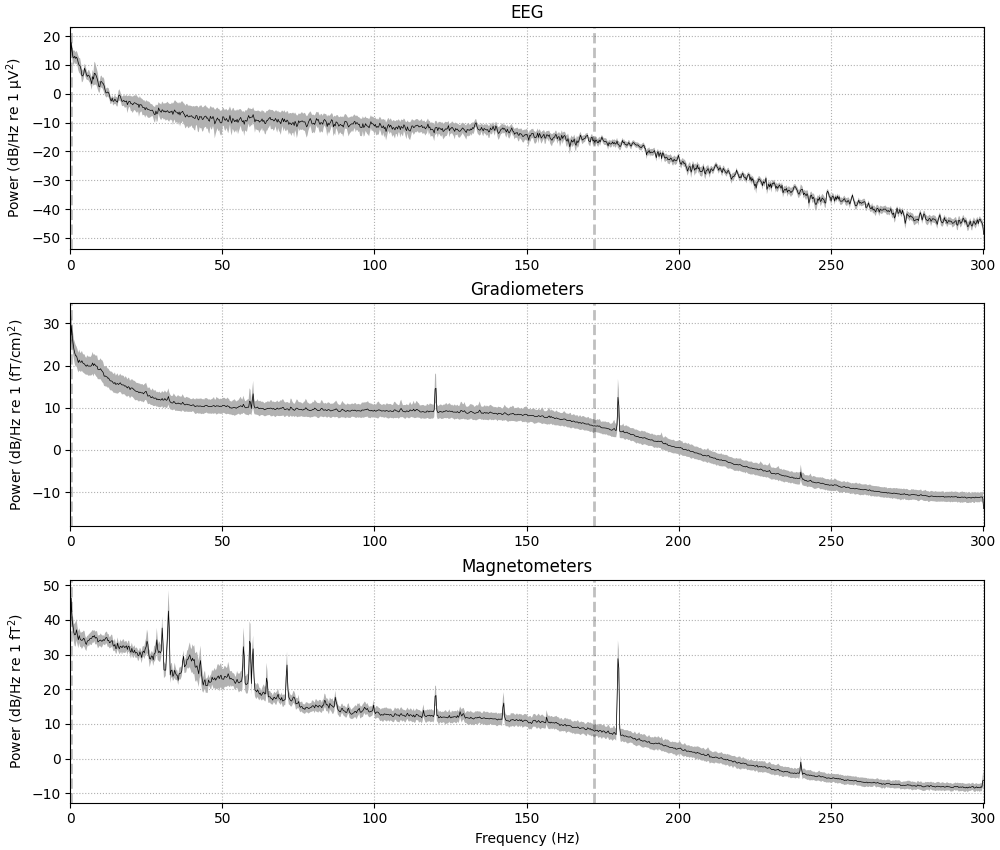```Effective window size : 3.410 (s)
```

If the data have been filtered, vertical dashed lines will automatically indicate filter boundaries. The spectrum for each channel type is drawn in its own subplot; here we’ve passed the `average=True` parameter to get a summary for each channel type, but it is also possible to plot each channel individually, with options for how the spectrum should be computed, color-coding the channels by location, and more. For example, here is a plot of just a few sensors (specified with the `picks` parameter), color-coded by spatial location (via the `spatial_colors` parameter, see the documentation of `plot` for full details):

```midline = ['EEG 002', 'EEG 012', 'EEG 030', 'EEG 048', 'EEG 058', 'EEG 060']
spectrum.plot(picks=midline)
```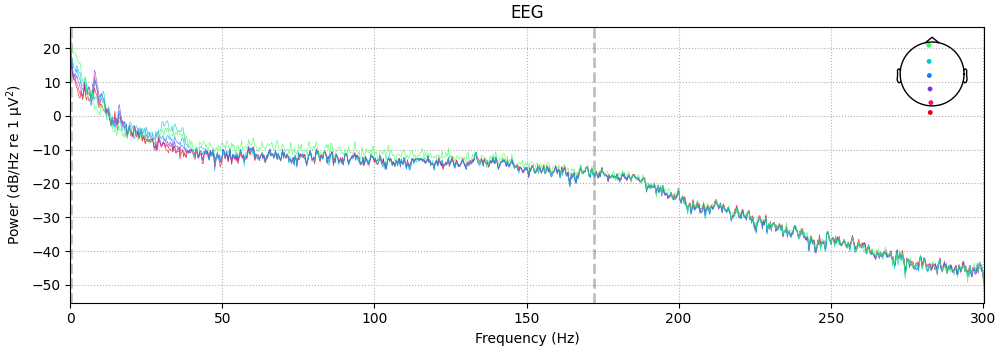It is also possible to plot spectral power estimates across sensors as a scalp topography, using the `Spectrum`’s `plot_topomap()` method. The default parameters will plot five frequency bands (δ, θ, α, β, γ), will compute power based on magnetometer channels (if present), and will plot the power estimates on a dB-like log-scale:

```spectrum.plot_topomap()
```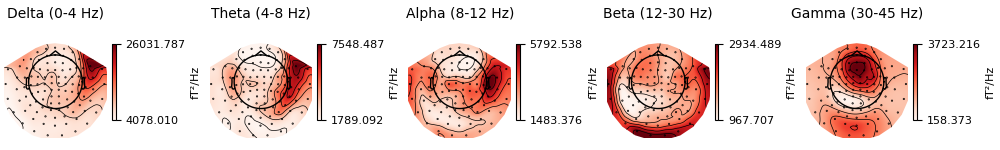Alternatively, you can plot the PSD for every sensor on its own axes, with the axes arranged spatially to correspond to sensor locations in space, using `plot_topo`:

```spectrum.plot_topo()
```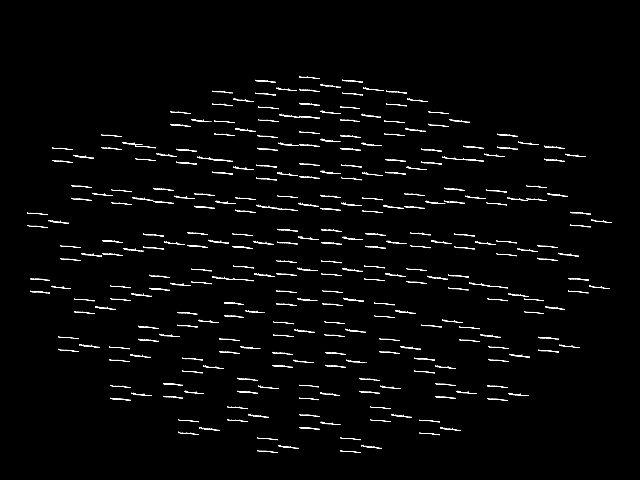This plot is also interactive; hovering over each “thumbnail” plot will display the channel name in the bottom left of the plot window, and clicking on a thumbnail plot will create a second figure showing a larger version of the selected channel’s spectral density (as if you had called `plot` with that channel passed as `picks`).

By default, `plot_topo` will show only the MEG channels if MEG channels are present; if only EEG channels are found, they will be plotted instead:

```spectrum.pick('eeg').plot_topo()
```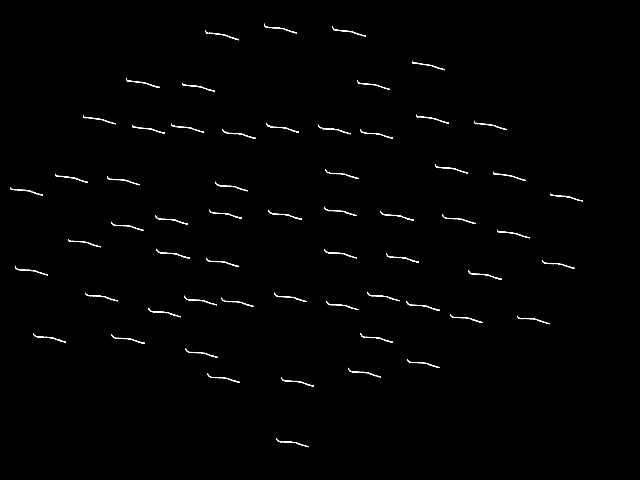```Removing projector <Projection | PCA-v1, active : False, n_channels : 102>
Removing projector <Projection | PCA-v2, active : False, n_channels : 102>
Removing projector <Projection | PCA-v3, active : False, n_channels : 102>
```

Note

Prior to the addition of the `Spectrum` class, the above plots were possible via:

```raw.plot_psd(average=True)
raw.plot_psd_topo()
raw.pick('eeg').plot_psd_topo()
```

(there was no `plot_topomap` method for `Raw`). The `plot_psd()` and `plot_psd_topo()` methods of `Raw` objects are still provided to support legacy analysis scripts, but new code should instead use the `Spectrum` object API.

## Plotting sensor locations from `Raw` objects#

The channel locations in a `Raw` object can be easily plotted with the `plot_sensors` method. A brief example is shown here; notice that channels in `raw.info['bads']` are plotted in red. More details and additional examples are given in the tutorial Working with sensor locations.

```raw.plot_sensors(ch_type='eeg')
```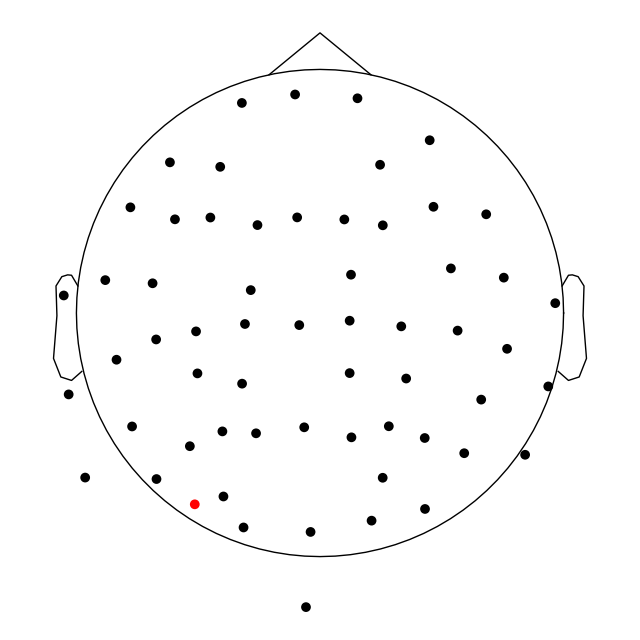## Plotting projectors from `Raw` objects#

As seen in the output of `mne.io.read_raw_fif` above, there are projectors included in the example `Raw` file (representing environmental noise in the signal, so it can later be “projected out” during preprocessing). You can visualize these projectors using the `plot_projs_topomap` method. By default it will show one figure per channel type for which projectors are present, and each figure will have one subplot per projector. The three projectors in this file were only computed for magnetometers, so one figure with three subplots is generated. More details on working with and plotting projectors are given in Background on projectors and projections and Repairing artifacts with SSP.

```raw.plot_projs_topomap(colorbar=True)
```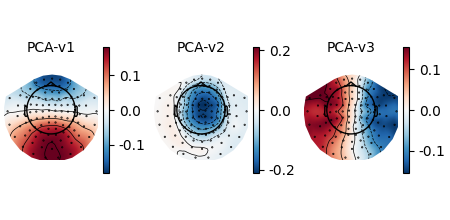Total running time of the script: ( 0 minutes 10.989 seconds)

Estimated memory usage: 212 MB

Gallery generated by Sphinx-Gallery